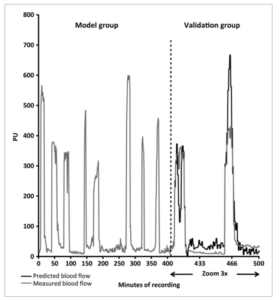## 23 Sep A novel model to predict cutaneous finger blood flow via finger and rectal temperaturesCarrillo AE, Cheung SS, Flouris AD. A novel model to predict cutaneous finger blood flow via finger and rectal temperatures. Microcirculation. 2011 Nov;18(8):670-6. doi: 10.1111/j.1549-8719.2011.00136.x. PMID: 21951311.

Abstract:

Objectives: To generate a model that predicts fingertip blood flow (BF(f) ) and to cross-validate it in another group of subjects.

Methods: We used fingertip temperature (T(f)), forearm temperature minus T(f) (T(For-f)), rectal temperature (T(re)), and their changes across time ((lag) T) to estimate BF(f). Ten participants (six male, four female) were randomly divided into “model” and “validation” groups. We employed a passive hot-cold water immersion protocol during which each participant’s core temperature increased and decreased by 0.5°C above/below baseline during hot/cold conditions, respectively. A hierarchical multiple linear regression analysis was introduced to generate models using temperature indicators and (lag) T (independent variables) obtained from the model group to predict BF(f) (dependent variable).

Results: Mean BF(f) (109.5 ± 158.2 PU) and predicted BF(f) (P-BF(f)) (111.4 ± 136.7 PU) in the model group calculated using the strongest (R(2) = 0.766, p < 0.001) prediction model [P-BF(f) =T(f) × 19.930 + (lag4) T(f) × 74.766 + (lag4) T(re) × 124.255 – 447.474] were similar (p = 0.6) and correlated (r = 0.880, p < 0.001). Autoregressive integrated moving average time-series analyses demonstrated a significant association between P-BF(f) and BF(f) (R(2) = 0.381; Ljung-Box statistic = 8.097; p < 0.001) in the validation group.

Conclusions: We provide a model that predicts BF(f) via two practical temperature indicators that can be implemented in both clinical and field settings.